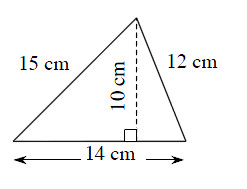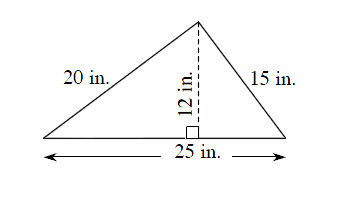### Home > MC1 > Chapter 10 > Lesson 10.1.3 > Problem10-37

10-37.

Find the perimeter and area of each triangle below.

The area of a triangle is equal to (0.5)(base)(height). The perimeter of a triangle is the sum of the lengths of all the sides.

1.The base of this triangle is $14$ cm and the height is $10$ cm.

Area$= \left(0.5\right)\left(14 \text{ cm}\right)\left(10 \text{ cm}\right) = 70$ sq cm
Can you find the perimeter?

1.If someone placed a string around the entire edge of this triangle, how long would the string have to be?

Perimeter $= 25 \text{ in.}+ 15 \text{ in.} + 20 \text{ in.} = 60$ inches
Can you find the area?